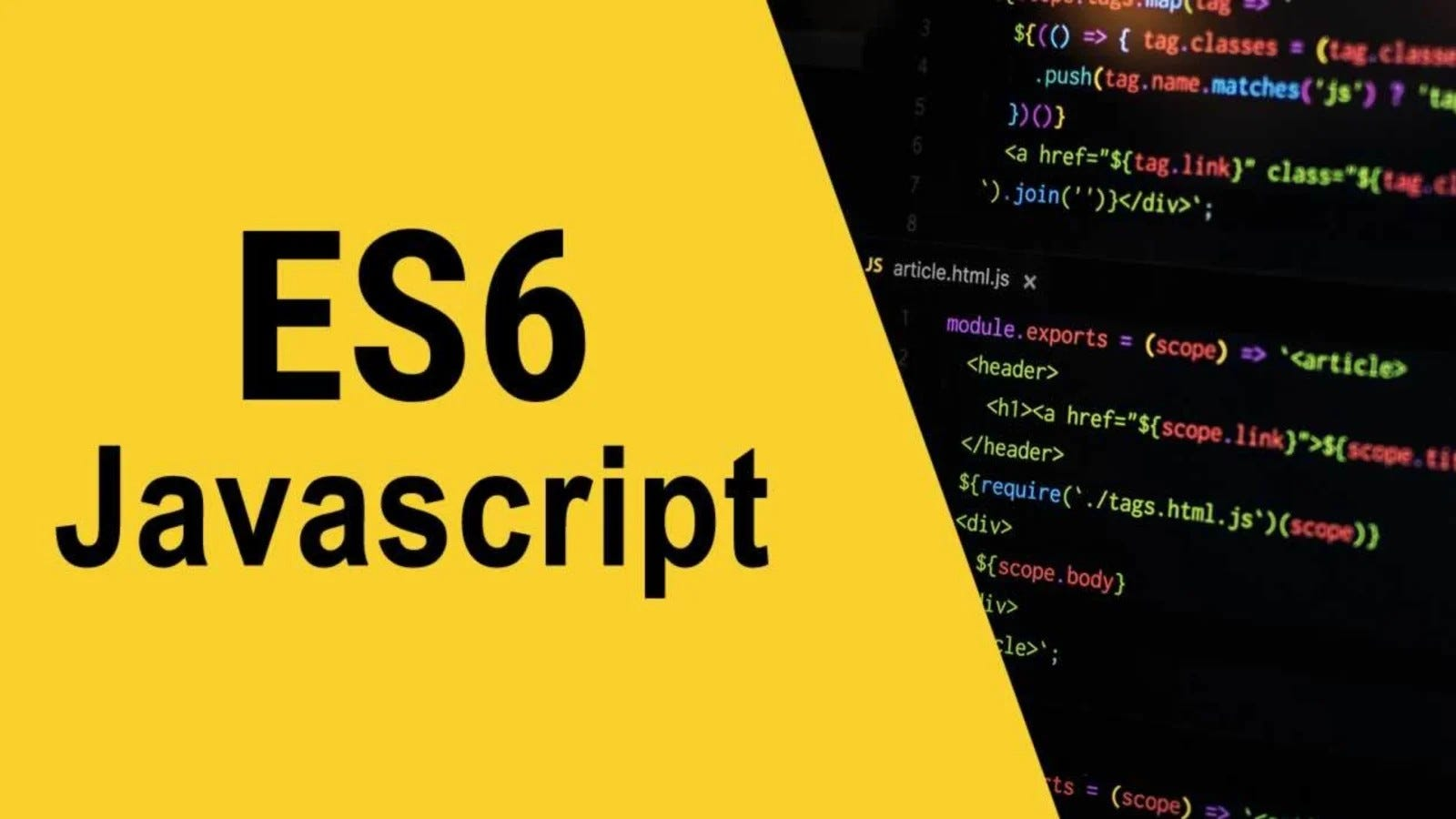# Some Important Factor of ES6ES6 Special

Building Blocks:
To be good at JavaScript, every beginner should start with understanding its building blocks. Building blocks are the smallest part of a big thing.
So the building blocks of JavaScript are Number, String, Boolean, Function, Object, and recently in Symbol is added as the Building block of JavaScript. But the list is not ended yet. In the building blocks things, there is null and undefined also.

Two types of Number:
The latest version of ECMAScript released a new numeric type, which is “BigInt”. Before then, we just knew the “Number” type. Like, integer, float, double are the number type. So, what is BigInt? Basically, in the Number type, there is a limitation. We cannot use the maximum limit of value in this type. And the max value is Number.MAX_SAFE_INTEGER which is 9007199254740991, well but what happens when we have to work with larger than this number? ECMA Script brings a new numeric type to solve this problem which is BigInt. To convert a normal number to a BigInt simply have to append ’n’ at the end of the number.

`// Numbertypeof(100) // output : "number"// BigInttypeof(100n) // output : "bigint"`

String.prototype.slice():
Slice is an interesting thing, Just forget about programming and think about a cake. When We have to eat a cake we don’t eat the whole cake at once. Rather, we cut that cake into a small piece and then eat that small piece. The same happens in String Splice Function. Whenever we want a specific portion of a string, we use the slice function. It has two parameters start index and end index. This function returns a new String also.

`const str = "I love JavaScript"let result = str.slice(7)// Output : "JavaScript"let result = str.slice(0, 6)// Output : "I love"`

String.prototype.split():
Split almost similar to slice, but the main difference is we have to give a separator or we can say the context where we want to make the split or division. So, This function takes two parameters String or regex pattern as a separator and number as a limit, and returns an array. Though both parameters are optional.

`const str = "I am a JavaScript Developer"str.split('JavaScript')// Output : ["I am a ", " Developer"]str.split('a')// Output : ["I ", "m ", " J", "v", "Script Developer"]str.split(' a ')// Output : ["I am", "JavaScript Developer"]str.split('a', 2) // With the limit Parameter// Output : ["I ", "m "]`

String.prototype.includes():
This function finds a particular ‘text’ from a selected string. Sometimes, we may need to check if any word exists in a string. Then we could use this function to find. This function takes to parameters. The first one is a String as a search string, which we want to find and the second one is the position as a number which is used to check in a particular position. And this includes function returns the result as a Boolean. It finds the word then It will return true, else it will return false.

`const str = 'JavaScript is a multi-paradigm, dynamic language';str.includes("JavaScript")// Output : truestr.includes("Javascript")  // Case Sensitive// Output : falsestr.includes("is", 11)    // Output : true// Second parameter defines the postion. It tries to find from 0 to the spacified postionstr.includes("is", 12)// Output : false`

Array.prototype.find():
This find method is self-explanatory. It finds a particular element of an array and returns the value. This function takes two arguments. The first one is the Callback function and the second one is ‘this’. The second parameter is optional. This function returns the matched element if it fits the condition, but if it didn’t it returns undefined.

`arr = [4,2,5,7,8]arr.find(element => element < 4)// Output : 2arr.find(element => element > 5)// Output : 7arr.find(element => element > 10)// Output : undefined// Cause, the condition is not fulfilled here.// Alternativefunction ifGreater(currentValue) {    return currentValue > 5}arr.find(ifGreater)// Output : 7`

Array.prototype.indexOf():
indexOf() function is used to find the index/position of a particular array element. Whenever you needed to find the position of a value or if you want to check if an element exists on the array, you can use this function to find it.
It takes two parameters, First one is the element which you wanted to find and the second one is the fromIndex parameter to find searching from a specific position. And It returns the position of the element in the array. If the element is not found it returns -1

`let array = ['I', 'Love', 'JavaScript']// index      0     1         2array.indexOf('JavaScript')// Output : 2array.indexOf('I')// Output : 0array.indexOf('javascript')// Output : -1// Case Sensitive so it's unable to find the value in the array`

Array.prototype.shift():

This shift() function is used to remove the first element from an array. And if the array is empty it returns undefined. This is a generic function, so it just can be called or applied to an Array. It doesn’t take parameters, but it returns the value that is removed from the Array.

`const array1 = [5, 10, 15, 20];array1.shift();// Output : 5// array1 = [10, 15, 20]const array2 = [];array2.shift();// Output : undefined`

Array.prototype.unshift():
unshift() do the exact opposite of shift(). Unshift function adds a new element to the existing array list as the first index. Even we can add multiple values or arrays to the first position.

## More from Satodhru Nondon

Junior Web Developer